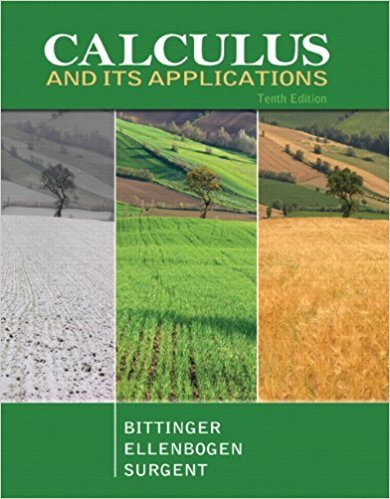×
×

# Solutions for Chapter 4.1: Antidifferentiation## Full solutions for Calculus and Its Applications | 10th Edition

ISBN: 9780321694331Solutions for Chapter 4.1: Antidifferentiation

Solutions for Chapter 4.1
4 5 0 373 Reviews
20
2
##### ISBN: 9780321694331

This expansive textbook survival guide covers the following chapters and their solutions. Since 88 problems in chapter 4.1: Antidifferentiation have been answered, more than 24312 students have viewed full step-by-step solutions from this chapter. Chapter 4.1: Antidifferentiation includes 88 full step-by-step solutions. This textbook survival guide was created for the textbook: Calculus and Its Applications, edition: 10. Calculus and Its Applications was written by and is associated to the ISBN: 9780321694331.

Key Calculus Terms and definitions covered in this textbook
• Augmented matrix

A matrix that represents a system of equations.

• Binomial theorem

A theorem that gives an expansion formula for (a + b)n

• Blocking

A feature of some experimental designs that controls for potential differences between subject groups by applying treatments randomly within homogeneous blocks of subjects

• Closed interval

An interval that includes its endpoints

• Definite integral

The definite integral of the function ƒ over [a,b] is Lbaƒ(x) dx = limn: q ani=1 ƒ(xi) ¢x provided the limit of the Riemann sums exists

• equation of a quadratic function

ƒ(x) = ax 2 + bx + c(a ? 0)

• Graph of a function ƒ

The set of all points in the coordinate plane corresponding to the pairs (x, ƒ(x)) for x in the domain of ƒ.

• Instantaneous velocity

The instantaneous rate of change of a position function with respect to time, p. 737.

• Interval

Connected subset of the real number line with at least two points, p. 4.

• Logarithmic re-expression of data

Transformation of a data set involving the natural logarithm: exponential regression, natural logarithmic regression, power regression

• Octants

The eight regions of space determined by the coordinate planes.

• Parameter

See Parametric equations.

• Polar coordinate system

A coordinate system whose ordered pair is based on the directed distance from a central point (the pole) and the angle measured from a ray from the pole (the polar axis)

• Rational numbers

Numbers that can be written as a/b, where a and b are integers, and b ? 0.

• Reflexive property of equality

a = a

• Remainder theorem

If a polynomial f(x) is divided by x - c , the remainder is ƒ(c)

• Repeated zeros

Zeros of multiplicity ? 2 (see Multiplicity).

• Semiminor axis

The distance from the center of an ellipse to a point on the ellipse along a line perpendicular to the major axis.

• Semiperimeter of a triangle

One-half of the sum of the lengths of the sides of a triangle.

• Tangent

The function y = tan x

×